# The Underlying Simulation Engine

All signal-integrity time-domain tools use iterative circuit-solution techniques pioneered by SPICE. An understanding of how SPICE operates therefore tells you a lot about how signal-integrity time-domain analysis algorithms behave (even if they are based on different software).

At its heart, SPICE is a guess-and-iterate algorithm. When simulating the performance of the simple circuit in Figure 13.2, SPICE performs the following steps.

Figure 13.2. According to the I-V tables, with v FET = v R1 = 1.65, more current would have to be flowing through the pull-up FET than through R1.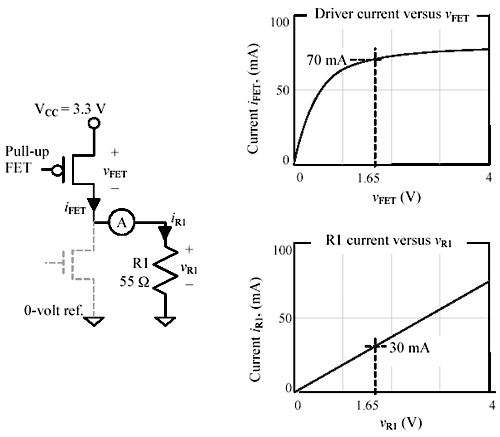1. Assume initial values for voltages v FET and v R1 .
2. Compute what currents flow as a consequence of the assumed voltages.
3. If the currents i 1 and i 2 are not equal, adjust the assumed voltages.
4. Repeat from step 2 until voltages converge on the correct values.

Figure 13.2 shows the I-V characteristics of the driver (which is pulling UP at the moment) and the resistive load. The I-V characteristics are crucial to the simulation process and must be supplied for each component.

Because the driver and load are wired in series, the simulator knows that at any given moment the voltage across the driver ( v FET ) plus the voltage across the load ( v R1 ) must equal the power supply voltage (V CC ). Imagine the algorithm starts by assuming v FET and v R1 each equal to half of V CC .

The driver output current associated with v FET = 1.65 V is given in the figure as 70 mA. The resistor current associated with v R1 = 1.65 V is given in the figure as 30 mA.

With this selection of voltages the driver current exceeds the resistor current by 40 mA, a clear violation of Kirchoff's current law, "The sum of currents into any node must equal zero", at node A. Therefore, the voltages must be wrong. You need to adjust the voltages in the direction of causing less driver current and more resistor current. This is done by lowering v FET and raising v R1 (the two are constrained to sum to V CC , so lowering one always raises the other).

How, you might ask, does the SPICE algorithm know in which direction to move v FET ? It doesn't; it merely tries both directions and then picks whichever direction seems to best improve the imbalance in current between the driver and the resistive load.

Figure 13.3 illustrates the result of lowering v FET and raising v R1 . The driver current is diminished, while the resistor current increased, bringing them closer to balance. If the iteration routine is smart (and SPICE is), it will keep adjusting the node voltages until it arrives at a point where the currents are acceptably equal. That's the gist of SPICE.

Figure 13.3. Decreasing v FET (and simultaneously increasing voltage v R1 ) decreases the current in the FET while increasing current in R1, bringing the circuit closer to balance.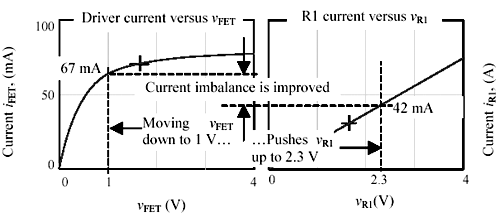Note that the iteration idea does not require that the I-V curves be linear, only that they be reasonably smooth. Also note that as long as the curves are monotonic, just about any searching strategy should lead to the correct answer after enough iterations. It might take a lot of iterations, but one of the great things about computers is that they have a lot of patience, so they can keep going around the try-and-evaluate loop over and over until they converge to an acceptable answer.

SPICE-like algorithms use mathematical techniques related to the Newton-Raphson algorithm to decide in which direction to move each guess value and how far to move it to obtain convergence. The Newton-Raphson algorithm is much faster than a simple binary search procedure, yielding very accurate answers within a small number of iterations for functions that are well behaved . For a circuit to be well behaved means that the I-V functions within it are monotonic and differentiable, plus a few other topological conditions. Most digital circuits, in the absence of feedback, have these properties. 

 An example of a very non-smooth I-V relationship is i ( v ) = sin(1/ v ). This function drives SPICE absolutely wild.

13.3.1 Evolving Forward

When you first engage a SPICE simulator, it attempts to derive steady-state initial circuit conditions. This is done by assuming all sources to be fixed at their initial values, all capacitances to be open circuits, and all inductances to be short circuits, and deriving according to the algorithm described previously the steady-state node voltages and currents everywhere. The algorithm is then ready to evolve forward , step-by-step, computing the state of the circuit at all future points in time. The evolution process, like everything else in SPICE, is iterative.

At each point after the algorithm has converged to a satisfactory set of node voltages for time t n , it evolves forward by a small time-step t to a new time t n +1 . The evolution process involves four steps:

• Time-varying input waveforms are advanced by one time-step.
• The voltage v C,k ( n ) stored on every capacitor C k at time t n is advanced  according to the current i C, k ( n ) then flowing through it:

 The evolution equations for capacitors and inductors are discrete-time, integral forms of the familiar relationships i c = C ( dv c / dt ) and v L = L ( di L / dt ).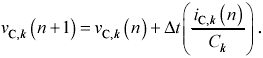• The current i L, m ( n ) flowing through every inductor L m at time t n is advanced according to the voltage v L, m ( n ) then impressed upon it: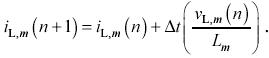• SPICE then accepts the new capacitor voltages (and new inductor currents) as fixed quantities and iterates the other node voltages and currents to find a complete solution for circuit behavior at time t n +1 .

13.3.2 Pitfalls of SPICE-Like Algorithms

One significant complicating factor for SPICE is circuit complexity. Our basic example had only two components , with one unknown value and one constraint. When modeling a real circuit, the SPICE algorithm must take into account hundreds or thousands of nodes and I-V relationships. The software to handle these features can become very complex, but it's not any different in philosophy from the simple two-element example in Figure 13.2. Regardless of the number of nodes, SPICE still operates by guesses and iteration.

From this brief description, I hope you can spot some of the weak points in the SPICE approach.

1. SPICE simulates the behavior of your circuit at discrete points in time. If the time-step size is set too coarsely, the results will be incorrect. If the time-step size is set too finely, the results will take too long to compute. Various forms of SPICE use different algorithms for optimizing the time-step size, algorithms that can be fooled by circuits with unusual or pathological configurations like narrow pulses , an extremely narrow resonance , or I-V relationships with narrow glitches. To handle these conditions, some versions of SPICE allow you to enforce a maximum step size so the algorithm can't skip over something important. Many engineers run their simulations twice, with two different step sizes. If the step size is sufficiently small, the results shouldn't vary.
2. Some versions of SPICE have a lower limit on the smallest permissible step size. If the limit is too coarse for your super-fast circuit, then that version of SPICE can't simulate your circuit. To circumvent this difficulty, multiply all your circuit capacitances by a factor of 1000, all your inductances by 1000, slow down all the time-domain inputs by a factor of 1000, and lengthen all lossless transmission lines by a factor of 1000. The resulting circuit will deliver exactly the same results as your original, only 1000 times more slowly. This trick may scale your circuit into a range that your version of SPICE can handle. If you are modeling lossy transmission lines, then you must scale the skin-effect resistance so that the new line, operating at a length 1000 times longer than the original, but at a frequency of 1/1000 the original, gives you the same skin-effect loss in dB. The same applies for dielectric loss.
3. At each step, SPICE must decide when to terminate its iteration. The termination decision is made by checking Kirchoff's current law against an arbitrary standard of what it means for the currents coming into and leaving each node to be "acceptably equal." This, and also the decision about when to automatically increase and decrease the time-step size, are what the SPICE variables TOL and REFTOL are all about. Check your documentation to make sure these quantities are set properly for your application. This is especially important when dealing with extremely small (or extremely large) voltages and currents.
4. The iteration routine may fail to converge. Especially on circuits that produce inductive spikes or have hard corners in their I-V curves, SPICE may fail to deliver an answer. In these cases sometimes the software knows it has done wrong and sometimes it does not. To cure inductive problems, shunt the inductor with a parasitic capacitance or a large resistor (like 1 M W ). If you are using I-V curves generated by a conglomeration of linear line segments, either use more segments or glue the segments together with quadratic segments calculated to maintain everywhere a continuous derivative.
5. SPICE operates only on the data you have supplied. If your parameter extraction efforts fail to properly account for all the significant parasitic elements in a circuit, the SPICE results will be incorrect. This problem can only be detected by comparing your results to real-world circuits.

SPICE is a powerful tool. Use it to quantify your analysis of circuit performance, but not to substitute for your understanding of circuit behavior. Only turn on SPICE after you have an idea of what a circuit is supposed to do.

To learn more about the strengths and weaknesses of SPICE, and especially how to mitigate inevitable difficulties with convergence, see the excellent book about SPICE by Ron Kielkowski  .

POINTS TO REMEMBER

• All signal-integrity time-domain analysis tools use simulation techniques pioneered by SPICE.
• Especially on circuits containing inductive spikes or hard corners in the I-V curves, SPICE may fail to converge.
• Some versions of SPICE have a lower limit on the smallest permissible step size.
• Check your documentation to make sure TOL and REFTOL are set properly for your application.
• If your parameter extraction efforts fail to properly account for all the significant parasitic elements in a circuit, SPICE results will be incorrect.

13.3.3 Transmission Lines

Most SPICE software packages include at least one built-in transmission line model. The most popular model is a simple, lossless, distortion-less transmission line. This lossless model is adequate for digital designs operating at risetimes of 1 ns and slower, on boards up to 25 cm (10 in.) across.

The SPICE transmission line modeling approach conceptually breaks the transmission line into a cascade of short sections, generating a linear two-port model for each section. (For a good overview of two-port models, see  ). Within each two-port section, SPICE uses two state variables to model the performance of that section. The state variables are (equivalent to) the instantaneous wave amplitudes in the forward and backward directions within each section ( v F n and v B n , respectively, Figure 13.4).

Figure 13.4. A two-port wave model for a transmission line separately accounts for signals propagating in both directions.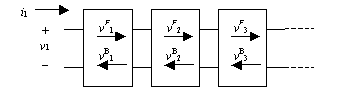What's nice about the two-port wave model is that SPICE can use simple shifting actions to represent the progress of the forward- and backward-propagating waves from one time-step to the next . Shifting actions are particularly simple to represent in software, as they can be implemented with rotating pointer rings. The only intensive calculations happen at the ends of the transmission line where the reflections take place. The forward-and-backward wave representation renders a lossless transmission-line model computationally efficient (although memory- intensive ).

The preceding description applies only the to modeling of linear, time-invariant, lossless transmission-line segments. For typical pcb traces up to 25 cm (10 in.) long, at risetimes of 1 ns or slower, the linear, time-invariant , lossless transmission-line model serves adequately well. At higher speeds or greater distances, the lossless assumption does not hold true. Furthermore, modifying the transmission-line model to incorporate a fixed loss per section doesn't help. The modeling of long traces used with very fast signals requires the use of a skin effect and dielectric-loss transmission-line model. Such models are significantly more complex and take a lot longer to run.

Section 5.2, "Limits to Attainable Distance," includes a very simple chart you can use to estimate pcb trace loss at any freqeuncy of interest. If the losses at the knee frequency of your logic amount to more than ten percent, then the accuracy of your simulations will noticeably benefit from using an advanced lossy line model. If you use a simple lossless transmission-line model, you'll miss the frequency-varying nature of the transmission-line effects. The step response of the line won't be correct, and your SPICE model may substantially underestimate jitter in the received waveform.

Advanced frequency-varying transmission-line models are significantly more complex than simple lossless transmission-line models. They are also fairly new, and may have bugs .  Check with your SPICE vendor to see if one is available (some vendors call it the W model).

 I hear persistent reports of convergence, tolerance, and memory problems associated with the W -element model.

When using any advanced, lossy-line time-domain model, keep in mind that the computation time varies with 1/ D t 2 , where D t represents the size of the simulation time-steps. Halving the step size doubles the number of time-steps and at the same time doubles the number of transmission-line segments usedquadrupling the simulation time. 

 The complexity of a frequency-domain simulator ( applicable only to linear systems) scales more slowly, growing only in proportion to -(ln D t )/ D t .

Also remember that the accuracy of the model is related to the transmission-line segment size. If you want better accuracy, use smaller sections. (Your SPICE system may or may not grant you access to this parameter).

POINTS TO REMEMBER

• The SPICE lossless transmission-line model is computationally efficient.
• For typical pcb traces up to 25 cm (10 in.) long, at risetimes of 1 ns or slower, a lossless transmission-line model serves adequately well. Higher speeds and greater distances require the use of a transmission-line model that accounts for the skin effect and dielectric-loss .
• Lossy transmission-line models take a lot longer to run.

It takes a lot of solid laboratory work to correlate your simulations with the real world. Do not underestimate the importance of this task or the possibility that one unanticipated flaw in your approach may render all your predictions meaningless. While you work, keep in mind these words of Thomas Alva Edison:

Being busy does not always mean real work. The object of all work is production or accomplishment and to either of these ends there must be forethought, system, planning, intelligence, and honest purpose, as well as perspiration. Seeming to do is not doing.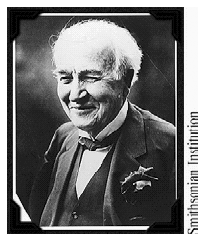When you first begin working with any simulator, set up some simple, low-frequency test circuits for which you can predict the exact response by hand calculations. Build the circuits in the simulation environment, and also build physical test circuits on the bench. Make sure your software predictions and physical measurements match within the tolerance limits of your equipment. Once you have debugged the first test circuit, move on to more complex (and faster) circuits.

For an initial test setup, I use a 20- foot piece of 50- W coax cable coupled to a 50- W square-wave generator with an adjustable risetime. Using a BNC T fitting I couple an extra 50- W terminator into the source, making the pulse generator into a 25- W source (50 W from the pulse generator in parallel with the external 50 W ). This produces a mild, easily predictable overshoot (33%) at the far end of the coax cable. With risetimes in the range of 10 to 100 ns, you should get excellent agreement between the software simulations and the real hardware measurements.

As you move up in the frequency range, both your probing technique and the construction of the test circuit become more critical. For test measurements at 500 MHz (1 ns rise time) and faster, I prefer SMA-type connectors in the test circuit and a resistive-input probe for the scope with a bandwidth at least three times the bandwidth of the signals under test.

Once you have a good correlation between simulation and reality, you can use your software tool with confidence.

If your manager does not understand the importance of doing these initial experiments, sneak into the lab and do them at night.

POINT TO REMEMBER

• When you first start working with any simulator, begin by setting up some simple, low-frequency test circuits for which you can predict the response by hand calculations.

13.3.5 Using SPICE Intelligently

SPICE does a superb job simulating the behavior of integrated circuits. It is quite capable of simulating the behavior of every transistor inside a logic circuit, even including logic functionality deep inside the chip. Unfortunately, if you try to do a complete circuit simulation in this manner, taking into account every transistor in every chip on a big board, the simulation may not complete within a reasonable amount of time.

If you need to simulate every transistor, there are no alternatives; you just have to turn on your computer and let it grind (sometimes for weeks). However, if your mission is merely to simulate ringing and crosstalk on connections between integrated circuits, consider using the modeling technique popularized by the I/O Buffer Information Specification (IBIS) standard. It is simpler and faster than a full transistor-level model. For ringing and crosstalk problems, it delivers suitably accurate answers.

The IBIS technique assumes foreknowledge of I-V tables and certain sampled waveforms representing your integrated circuits. It also assumes the calculations for each net are separable . That is, it assumes no knowledge of coincidental switching or of functionality within each chip. The technique merely steps through each net, trying each driver, calculating the received waveforms for both high and low transitions at all the receiver locations. This set of received waveforms is then analyzed to extract worst-case values for overshoot, ringback, settling time, and crosstalk on each net.High-Speed Signal Propagation[c] Advanced Black Magic
ISBN: 013084408X
EAN: N/A
Year: 2005
Pages: 163